# Polynomial Chapter 2 Class 9 Exercise 2.4 and 2.5

In this page we have NCERT Solutions for Class 9 Maths Chapter 2 Polynomial for Exercise 2.4 and 2.5 . Hope you like them and do not forget to like , social share and comment at the end of the page.

## Chapter 2 Exercise 2.4

Question 1
Determine which of the following polynomials has (x+ 1) a factor:
(i)$x^3+x^2+x+ 1$
(ii)$x^4+x^3+x^2+x+ 1$
(iii)$x^4+ 3x^3+ 3x^2+x+ 1$
(iv)$x^3-x^2- (2+ \sqrt {2}) x + \sqrt {2}$
Solution
We know from remainder theorem, if (x-a) is a factor of polynomial p(x) then p(a)=0
(i) If (x+ 1) is a factor of $p(x) =x^3+x^2+x+ 1$
Then p (-1) must be zero.
Here,$p(x) =x^3+x^2+x+ 1$
$p (-1) = (-1)^3+ (-1)^2+ (-1) + 1$
$= -1 + 1 - 1 + 1 = 0$
Hence,x+ 1 is a factor of this polynomial

(ii) If (x+ 1) is a factor of $p(x) =x^4+x^3+x^2+x+ 1$
Then p (-1) must be zero.
$p (-1) = (-1)^4+ (-1)^3+ (-1)^2+ (-1) + 1$
= 1
As, $p (-1) \ne 0$
Hence,x+ 1 is not a factor of this polynomial

(iii)If (x+ 1) is a factor of polynomial $p(x) =x^4+ 3x^3+ 3x^2+x+ 1$
Then p (- 1) must be 0.
$p (-1) = (-1)^4+ 3(-1)^3+ 3(-1)^2+ (-1) + 1$
= 1
As, $p (-1) \ne 0$
Hence,x+ 1 is not a factor of this polynomial.

(iv) If (x+ 1) is a factor of polynomial
$p(x) =x^3-x^2- (2+ \sqrt {2}) x + \sqrt {2}$
Then p (- 1) must be 0.
$p (-1) = (-1)^3- (-1)^2- (2+ \sqrt {2})(-1)+\sqrt {2}$
$= -1 - 1+ 2 + \sqrt {2} + \sqrt {2}$
$=2\sqrt {2}$
As, $p(-1) \ne 0$
Hence,x+ 1 is not a factor of this polynomial.

Question 2.
Use the Factor Theorem to determine whether g(x) is a factor of p(x) in each of the following cases:
(i)$p(x) = 2x^3+x^2- 2x- 1$,$g(x) =x+ 1$
(ii)$p(x) =x^3+ 3x^2+ 3x+ 1$,$g(x) =x+ 2$
(iii) $p(x) =x^3- 4x^2+x+ 6$,$g(x) =x- 3$
Solution
(i) If $g(x) =x+ 1$ is a factor of given polynomial p(x)
Then, $p(- 1)$ must be zero.
$p(x) = 2x^3+x^2- 2x- 1$
$p(- 1) = 2(-1)^3+ (-1)^2- 2(-1) - 1$
$= 2(- 1) + 1 + 2 - 1 = 0$
Hence,g(x) =x+ 1 is a factor of given polynomial.

(ii) If $g(x) =x+ 2$ is a factor of given polynomial p(x)
Then, $p(-2)$ must be 0.
$p(x) =x^3+3x^2+ 3x+ 1$
$p(-2) = (-2)^3+ 3(- 2)^2+ 3(- 2) + 1$
$= -8 + 12 - 6 + 1$
$=-1$
As, $p(-2) \ne 0$
Therefore, $g(x) =x+ 2$ is not a factor of given polynomial.

(iii) If $g(x) =x- 3$ is a factor of given polynomial $p(x)$
Then, p(3) must be 0.
$p(x) =x^3- 4x^2+x+ 6$
$p(3) = (3)^3- 4(3)^2+ 3 + 6$
$= 27 - 36 + 9 = 0$
Hence,$g(x) =x- 3$ is a factor of given polynomial.

Question 3.
Find the value of k, if x- 1 is a factor of p(x) in each of the following cases:
(i)$p(x) =x^2+x+k$
(ii)$p(x) = 2x^2+kx+ \sqrt{2}$
(iii)$p(x) =kx^2- \sqrt{2} x+ 1$
(iv)$p(x) =kx^2-3x+k$
Solution
(i) If x- 1 is a factor of polynomial $p(x) =x^2+x+k$, then
$p(1) = 0$
$(1)^2+ 1 +k= 0$
$2 +k= 0$
$k= - 2$
So, value of k is -2.

(ii) If x- 1 is a factor of polynomial $p(x) = 2x^2+kx+ \sqrt{2}$, then
$p(1) = 0$
$2(1)^2+k(1) + \sqrt{2}= 0$
$k= -2 - \sqrt{2}= -(2 + \sqrt{2})$
So, value of k is $- (2 + \sqrt{2})$.

(iii) If x- 1 is a factor of polynomial $p(x) =kx^2- \sqrt{2}x+ 1$, then
$p (1) = 0$
$k(1)^2- \sqrt{2}(1) + 1= 0$
$k= \sqrt{2}-1$
So, value of k is $\sqrt{2}-1$

(iv) If x- 1 is a factor of polynomial $p(x) =kx^2-3x+k$, then
$p(1) = 0$
$k(1)^2+ 3(1) +k= 0$
$k- 3+k= 0$
$k= \frac {3}{2}$
So, value of k is $\frac {3}{2}$

Question 4
Factorise:
(i) $12x^2+ 7x+ 1$
(ii) $2x^2+ 7x+ 3$
(iii)$6x^2+ 5x- 6$
(iv)$3x^2-x- 4$
Solution
Here we would be using splitting the middle term to factorize the polynomial
To factorise $ax^2 +bx +c$, we should write b as the sum of two numbers whose product is ac
(i) $12x^2+ 7x+ 1$
Here a=12, c=1 and b=7 So $b=7=3+4$ ,$ac=12 \times 1=12= 3 \times 4$
$12x^2+ 7x+ 1$
$=12x^2+4x+ 3x+ 1$
$= 4x(3x+ 1) + 1 (3x+ 1)$
$= (3x+1) (4x+ 1)$

(ii) $2x^2+ 7x+ 3$
Here a=2, c=3 and b=7 So $b=7=6+1$ ,$ac= 2 \times 3=6 = 6 \times 1$
$= 2x^2+ 6x+x+ 3$
$= 2x(x+ 3) + 1 (x+ 3)$
$=(x+ 3) (2x+ 1)$

(iii) $6x^2+ 5x- 6$
Here a=6, c=-6 and b=5 So, $b=5=9 + (-4)$ ,$ac= 6 \times (-6) =-36= 9 \times (-4)$
$=6x^2+ 9x- 4x- 6$
$= 3x(2x+ 3) - 2 (2x+ 3)$
$= (2x+ 3) (3x- 2)$

(iv) $3x^2-x- 4$
Here a=3, c=-4 and b=-1 So, $b=-1=-4+3$, $ac= 3 \times (-4) =-12=(-4) \times 3$
$=3x^2- 4x+ 3x- 4$
$=x(3x- 4) + 1 (3x- 4)$
$= (3x- 4) (x+ 1)$

Question 5.
Factorise:
(i) $x^3- 2x^2-x+ 2$
(ii) $x^3- 3x^2- 9x- 5$
(iii) $x^3+ 13x^2+ 32x+ 20$
(iv) $2y^3+y^2- 2y- 1$
Solution
These are cubic polynomials and can be factorized using combination of long division method , remainder theorem and split middle term method
(i) Let $p(x) =x^3- 2x^2-x+ 2$
Factors of 2 are ±1 and ± 2
By trial method, we find that
$p(-1)= (-1)^3- 2(-1)^2- (-1)+ 2=0$
So,(x+1) is factor of p(x)
. Now using the Long division method , we can find the quotient as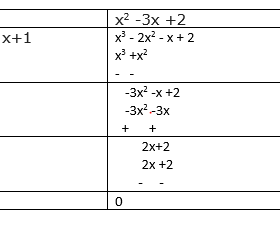Now, $Dividend = Divisor \times Quotient+ Remainder$
$x^3- 2x^2-x+ 2=(x+1) (x^2- 3x + 2)$
Factorizing the second part by split middle term method
$= (x+1) (x^2- x - 2x + 2)$
$= (x+1) [x(x-1) -2(x-1)]$
$= (x+1) (x-1) (x+2)$

(ii) Let $p(x) =x^3- 3x^2- 9x- 5$
Factors of 5 are ±1 and ±5
By trial method, we find that
$p(5)= 5^3- 3 \times 5^2- 9 \times 5- 5=0$
So,(x-5) is factor of p(x)
Now using the Long division method , we can find the quotient as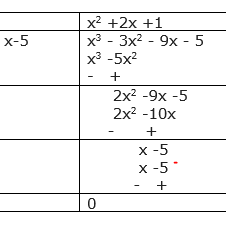Now, $Dividend = Divisor \times Quotient+ Remainder$
$x^3- 3x^2- 9x- 5=(x-5) (x^2+ 2x + 1)$
Factorizing the second part by split middle term method
$= (x-5) (x^2+ x + x + 1)$
$=(x-5){x(x+1) +1(x+1)}$
$=(x-5)(x+1)(x+1)$

(iii) Let $p(x) =x^3+ 13x^2+ 32x+ 20$
Factors of 20 are ±1, ±2, ±4, ±5, ±10 and ±20
By trial method, we find that
$p(-1)= (-1)^3+ 13(-1)^2+ 32(-1)+ 20=0$
So,(x+1) is factor ofp(x)
Now using the Long division method , we can find the quotient as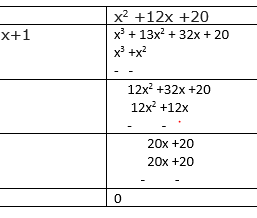Now, Dividend = Divisor \times Quotient+ Remainder
$x^3+ 13x^2+ 32x+ 20=(x+1) (x^2+ 12x + 20)$
Factorizing the second part by split middle term method
$= (x+1) (x^2+ 2x+ 10x + 20)$
$=(x-5)[x(x+2) +10(x+2)]$
$=(x-5)(x+2)(x+10)$

(iv) Let $p(y) = 2y^3+y^2- 2y- 1$
Factors of ab = 2 \times (-1) = -2 are ±1 and ±2
By trial method, we find that
$p(1)= 2(1)^3+(1)^2- 2(1)- 1=0$
So,(y-1) is factor of p(y)
Now using the Long division method , we can find the quotient as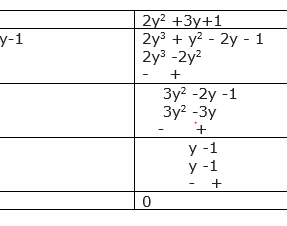Now, $Dividend = Divisor \times Quotient+ Remainder$
$2y^3+y^2- 2y- 1=(y-1) (2y^2+ 3y + 1)$
Factorizing the second part by split middle term method
$=(y-1)(2y^2+ 2y+ y + 1)$
$=(y-1)[2y(y+1) +1(y+1)]$
$=(y-1)(2y+1)(y+1)$

## Chapter 2 Exercise 2.5

Question 1
Use suitable identities to find the following products:
(i) $(x+ 4) (x+ 10)$
(ii) $(x+ 8) (x- 10)$
(iii) $(3x+ 4) (3x- 5)$
(iv) $(y^2+ \frac {3}{2}) (y^2- \frac {3}{2})$
(v) $(3 - 2x) (3 + 2x)$
Solution
(i)Using identity, $(x+ a) (x+ b) =x^2+ (a + b)x+ ab$
Here a = 4 and b = 10
Therefore,
$(x+ 4) (x+ 10) =x^2+ (4 + 10)x+ (4 \times 10)$
$=x^2+ 14x+ 40$

(ii) $(x+ 8) (x -10)$
Using identity, $(x+ a) (x+ b) =x^2+ (a + b)x+ ab$
Here, a = 8 and b = -10
Therefore,
$(x+ 8) (x -10) =x^2+ {8 +(- 10)}x+ {8 \times (- 10)}$
$=x^2- 2x- 80$

(iii) $(3x+ 4) (3x - 5)$
Using identity, $(x+ a) (x+ b) =x^2+ (a + b)x+ ab$
Here,x= 3x,a = 4 and b = -5
Therefore,
$(3x+ 4) (3x- 5) = (3x)^2+ {4 + (-5)}3x+ {4 \times (-5)}$
$= 9x^2+ 3x (4 - 5) - 20$
$= 9x^2- 3x- 20$

(iv) $(y^2+ \frac {3}{2}) (y^2- \frac {3}{2})$
Using identity, $(x+y) (x-y) =x^2-y^2$
Here,$x=y^2$ and $y= \frac {3}{2}$
Therefore,
$(y^2+ \frac {3}{2}) (y^2- \frac {3}{2}) = (y^2)^2- (\frac {3}{2})^2$
$=y^4- \frac {9}{4}$

(v) $(3 - 2x) (3 + 2x)$
Using identity, $(x+y) (x-y) =x^2-y^2$
Here,x= 3 andy= 2x
Therefore,
$(3 - 2x) (3 + 2x) = 3^2- (2x)^2$
$=9- 4x^2$

Question 2
Evaluate the following products without multiplying directly:
(i) $103 \times 107$
(ii) $95 \times 96$
(iii) $104 \times 96$
Solution
(i) $103 \times 107$ can be written as
$= (100+ 3) (100+ 7)$
Using identity, $(x+ a) (x+ b) =x^2+ (a + b)x+ ab$
Here,x= 100, a = 3 and b = 7
Therefore,
$103 \times 107 = (100+ 3) (100+ 7) = (100)^2+ (3 + 7)10 + (3 \times 7)$
$= 10000+ 100 + 21$
$= 10121$
(ii) $95 \times 96 = (90 + 5) (90 + 4)$
Using identity, $(x+ a) (x+ b) =x^2+ (a + b)x+ ab$
Here,x= 90, a = 5 and b = 4
Therefore,

$95 \times 96 = (90 + 5) (90 + 4) = 90^2+ 90(5 + 6) + (5 \times 6)$
$= 8100 + (11 \times 90) + 30$
$= 8100 + 990 + 30 = 9120$

(iii) $104 \times 96 = (100 + 4) (100 - 4)$
Using identity, $(x+y) (x-y) =x^2-y^2$
Here,x= 100 andy= 4
Therefore,
$104 \times 96 = (100 + 4) (100 - 4) = (100)^2-(4)^2= 10000 - 16 = 9984$

Question 3
Factorise the following using appropriate identities:
(i) $9x^2+ 6xy+y^2$
(ii) $4y^2- 4y+ 1$
(iii) $x^2- \frac {y^2}{100}$
Solution
(i)$9x^2+ 6xy + y^2 = (3x)^2+ (2 \times 3x \times y) + y^2$
Using identity, $(a + b)^2= a^2+ 2ab + b^2$
Here, a = 3x and b =y
$9x^2+ 6xy + y^= (3x)^2+ (2 \times 3x \times y) + y^2= (3x + y)^2= (3x + y) (3x + y)$

(ii)$4y^2- 4y + 1 = (2y)^2- (2 \times 2y \times 1) + 1^2$
Using identity, $(a - b)^2= a^2- 2ab + b^2$
Here, a =2y and b =1
$4y^2- 4y + 1 = (2y)^2- (2 \times 2y \times 1) + 1^2= (2y - 1)^2= (2y - 1) (2y - 1)$

(iii)$x^2- \frac {y^2}{100} = x^2- (\frac {y}{10})^2$
Using identity, $a^2- b^2= (a + b) (a - b)$
Here, a =x and b =(y/10)
$x^2- \frac {y^2}{100} = x^2- (\frac {y}{10})^2= (x- \frac {y}{10}) (x+ \frac {y}{10})$

Question 4
Expand each of the following, using suitable identities:
(i) $(x+ 2y+ 4z)^2$
(ii) $(2x-y+z)^2$
(iii) $(-2x+ 3y+ 2z)^2$
(iv) $(3a- 7b-c)^2$
(v) $(-2x+ 5y- 3z)^2$
(vi) $[\frac {1}{4}a - \frac {1}{2}b + 1]^2$
We will be using the identity
$(a + b +c)^2= a^2+ b^2+ c^2+ 2ab + 2bc + 2ca$

(i) $(x+ 2y+ 4z)^2$
Here, a =x, b = 2yand c = 4z
$(x+ 2y+ 4z)^2=x^2+(2y)^2+ (4z)^2+ (2 \times x \times 2y) + (2 \times 2y \times 4z) + (2 \times 4z \times x)$
$=x^2+ 4y^2+ 16z^2+ 4xy + 16yz + 8xz$

(ii)$(2x-y+z)^2$
Here, a =2x, b = -y and c =z
$(2x-y+z)^2=(2x)^2+ (-y)^2+ z^2+ (2 \times 2x \times -y)+ (2 \times -y \times z)+ (2 \times z \times 2x)$
$=4x^2+ y^2+ z^2- 4xy- 2yz+ 4xz$

(iii) $(-2x+ 3y+ 2z)^2$
Here, a =-2x, b = 3y and c =2z
$(-2x + 3y + 2z)^2=(-2x)^2+ (3y)^2+ (2z)^2+ (2 \times -2x \times 3y)+ (2 \times 3y \times 2z)+ (2 \times 2z \times -2x)$
$=4x^2+ 9y^2+ 4z^2- 12xy+ 12yz- 8xz$

(iv) $(3a- 7b-c)^2$
Here, a =3a, b = -7b and c =-c
$(3a - 7b - c)^2=(3a)^2+ (-7b)^2+ (-c)^2+ (2 \times 3a \times -7b)+ (2 \times -7b \times -c)+ (2 \times -c \times 3a)$
$=9a^2+ 49b^2+ c^2- 42ab+ 14bc- 6ac$

(v) $(-2x+ 5y- 3z)^2$
Here, a =-2x, b =5y and c =-3z
$(-2x + 5y - 3z)^2=(-2x)^2+ (5y)^2+ (-3z)^2+ (2 \times -2x \times 5y)+ (2 \times 5y \times -3z)+ (2 \times -3z \times -2x)$
$=4x^2+ 25y^2+ 9z^2- 20xy- 30yz+ 12xz$

(vi) $[\frac {1}{4}a - \frac {1}{2}b + 1]^2$
Here, $a = \frac {1}{4} a$, $b = \frac {-1}{2}b$ and c = 1
$[\frac {1}{4}a - \frac {1}{2}b + 1]^2=(\frac {1}{4}a)^2+ (\frac {-1}{2}b)^2+1^2+ (2 \times \frac {1}{4}a \times \frac {-1}{2}b)+ (2 \times \frac {-1}{2}b \times 1)+ (2 \times 1 \times \frac {1}{4}a)$
$=\frac {1}{16} a^2 + \frac {1}{4} b^2 +1 - \frac {1}{4} ab - b + \frac {1}{2}a$

Question 5
Factorise:
(i) $4x^2+ 9y^2+ 16z^2+ 12xy- 24yz- 16xz$
(ii) $2x^2+y^2+ 8z^2- 2\sqrt {2} xy+ 4 \sqrt {2} yz- 8xz$

Solution

(i) $4x^2+ 9y^2+ 16z^2+ 12xy- 24yz- 16xz$
Using identity, $(a + b +c)^2= a^2+ b^2+ c^2+ 2ab + 2bc + 2ca$
$4x^2+ 9y^2+ 16z^2+ 12xy - 24yz - 16xz$
$= (2x)^2+ (3y)^2+ (-4z)^2+ (2 \times 2x \times 3y) + (2 \times 3y \times -4z) + (2 \times -4z \times 2x)$
$=(2x + 3y - 4z)^2$
$=(2x + 3y - 4z) (2x + 3y - 4z)$

(ii) $2x^2+y^2+ 8z^2- 2\sqrt {2} xy+ 4 \sqrt {2} yz- 8xz$
Using identity, $(a + b +c)^2= a^2+ b^2+ c^2+ 2ab + 2bc + 2ca$
$2x^2+y^2+ 8z^2- 2\sqrt {2} xy+ 4 \sqrt {2} yz- 8xz$
$= (-\sqrt{2} x)^2+ (y)^2+ (2\sqrt {2}z)^2+ (2 \times -\sqrt {2} x \times y) + (2 \times y \times 2 \sqrt {2}z) + (2 \times 2\sqrt {2} z \times -\sqrt {2}x)$
$=(-\sqrt {2}x+ y+2\sqrt {2}z)^2$
$=(-\sqrt {2}x+ y+2\sqrt {2}z)(-\sqrt {2}x+ y+2\sqrt {2}z)$

Question 6
Write the following cubes in expanded form:
(i) $(2x + 1)^3$
(ii) $(2a - 3b)^3$
(iii) $[\frac {3}{2} x+ 1]^3$
(iv) $[x- \frac {2}{3}y]^3$
Solution
We will be using the identity
$(a + b)^3= a^3+ b^3+ 3ab (a + b)$
$(a - b)^3= a^3- b^3- 3ab (a - b)$

(i) $(2x+ 1)^3$
$=(2x)^3+ 1^3+(3 \times 2x \times 1) (2x + 1)$
$= 8x^3+ 1 +6x (2x + 1)$
$=8x^3+12x^2+6x+ 1$

(ii) $(2a - 3b)^3$
$= (2a)^3- (3b)^3- (3 \times 2a \times 3b) (2a - 3b)$
$= 8a^3- 27b^3- 18ab (2a - 3b)$
$=8a^3- 27b^3- 36a^2b+ 54ab^2$

(iii) $[\frac {3}{2} x+ 1]^3$
$= (\frac {3}{2} x)^3+ 1^3+(3 \times \frac {3}{2} x \times 1) (\frac {3}{2} x + 1)$
$= \frac {27}{8}x^3+ 1 +\frac {9}{2} x (\frac {3}{2} x + 1)$
$=\frac {27}{8} x^3+ 1 +\frac {27}{4} x^2+ \frac {9}{2} x$
$=\frac {27}{8}x^3+\frac {27}{4}x^2+ \frac {9}{2} x+ 1$

(iv) $[x- \frac {2}{3}y]^3= (x)^3- (\frac {2}{3}y)^3- (3 \times x \times \frac {2}{3}y) (x - \frac {2}{3}y)$
$= x^3- \frac {8}{27}y^3- 2xy (x - \frac {2}{3}y)$
$=x^3- \frac {8}{27}y^3- 2x^2y+ \frac {4}{3}xy^2$

Question 7
Find the value of the following using suitable identities:
(i) $(99)^3$
(ii) $(102)^3$
(iii) $(998)^3$
We will be using the identity
$(a + b)^3= a^3+ b^3+ 3ab (a + b)$
$(a - b)^3= a^3- b^3- 3ab (a - b)$

(i) $(99)^3= (100 - 1)^3$
$= (100)^3- 1^3- (3 \times 100 \times 1) (100 - 1)$
$= 1000000 - 1 - 300(100 - 1)$
$=1000000 - 1 - 30000 + 300$
$= 970299$

(ii) $(102)^3= (100 + 2)^3$
$= (100)^3+ 2^3+ (3 \times 100 \times 2) (100 + 2)$
$= 1000000+ 8+ 600(100 + 2)$
$=1000000+ 8+ 60000 + 1200$
$= 1061208$

(iii) $(998)^3$
$= (1000)^3- 2^3- (3 \times 1000 \times 2) (1000 - 2)$
$= 100000000 - 8 - 6000(1000 - 2)$
$=100000000 - 8- 600000 + 12000$
$= 994011992$

Question 8
Factorise each of the following:
(i) $8a^3+ b^3+ 12a^2b + 6ab^2$
(ii) $8a^3- b^3- 12a^2b + 6ab^2$
(iii) $27 - 125a^3- 135a + 225a^2$
(iv) $64a^3- 27b^3- 144a^2b + 108ab^2$
(v) $27p^3- \frac {1}{216} - \frac {9}{2} p^2 + \frac {1}{4} p$

Solution
(i)$8a^3+ b^3+ 12a^2b + 6ab^2$
Using identity, $(a + b)^3= a^3+ b^3+ 3a^2b+ 3ab^2$
$8a^3+ b^3+ 12a^2b + 6ab^2$
$=(2a)^3+ b^3+3(2a)^2b+3(2a) (b)^2$
$= (2a + b)^3$
$= (2a + b) (2a + b) (2a + b)$

(ii) $8a^3- b^3- 12a^2b + 6ab^2$
Using identity, $(a - b)^3= a^3- b^3- 3a^2b + 3ab^2$
$8a^3- b^3- 12a^2b + 6ab^2=(2a)^3- b^3-3(2a)^2b+3(2a) (b)^2$
$= (2a - b)^3$
$= (2a - b) (2a - b) (2a - b)$

(iii) $27 - 125a^3- 135a + 225a^2$
Using identity, $(a - b)^3= a^3- b^3- 3a^2b + 3ab^2$
$27 - 125a^3- 135a + 225a^2=3^3- (5a)^3-3(3)^2(5a)+3(3) (5a)^2$
$= (3 - 5a)^3$
$=(3 - 5a) (3 - 5a) (3 - 5a)$

(iv) $64a^3- 27b^3- 144a^2b + 108ab^2$
Using identity, $(a - b)^3= a^3- b^3- 3a^2b + 3ab^2$
$64a^3- 27b^3- 144a^2b + 108ab^2=(4a)^3- (3b)^3-3(4a)^2(3b)+3(4a) (3b)^2$
$= (4a - 3b)^3$
$= (4a - 3b) (4a - 3b) (4a - 3b)$

(v)$27p^3- \frac {1}{216} - \frac {9}{2} p^2 + \frac {1}{4} p$

Using identity, $(a - b)^3= a^3- b^3- 3a^2b + 3ab^2$
$27p^3- \frac {1}{216} - \frac {9}{2} p^2 + \frac {1}{4} p$
$=(3p)^3- (\frac {1}{6})^3-3(3p)^2(\frac {1}{6})+3(3p) (\frac {1}{6})^2$
$= (3p - \frac {1}{6})^3$
$= (3p - \frac {1}{6}) (3p - \frac {1}{6}) (3p - \frac {1}{6})$

Question 9
Verify the below identities
(i)$x^3+y^3= (x+y) (x^2-xy+ y^2)$
(ii)$x^3-y^3= (x-y) (x^2+xy+ y^2)$
Solution

(i)$x^3+y^3= (x+y) (x^2-xy+ y^2)$
We know that,
$(x + y)^3= x^3+ y^3+3xy (x + y)$
Taking $3xy (x + y)$ on another side, we get
$x^3+ y^3= (x + y)^3-3xy (x + y)$
Taking (x + y) common factor
$= (x + y) [(x + y)^2-3xy]$
$= (x + y)[(x^2+ y^2+ 2xy) -3xy]$
$= (x + y)(x^2+ y^2- xy)$

(ii)$x^3-y^3= (x-y) (x^2+xy+ y^2)$
We know that,
$(x - y)^3= x^3- y^3-3xy(x - y)$
$x^3- y^3= (x - y)^3+3xy(x - y)$
Taking $(x-y)$ common factor
$= (x - y)[(x - y)^2+3xy]$
$= (x - y)[(x^2+ y^2- 2xy)+3xy]$
$= (x + y)(x^2+ y^2+ xy)$
Question 10
Factorise each of the following:
(i) $27y^3+ 125z^3$
(ii) $64m^3- 343n^3$
Solution
(i) $27y^3+ 125z^3$
Using identity proved in the above question, $x^3+y^3= (x+y) (x^2-xy+ y^2)$
$27y^3+ 125z^3=(3y)^3+ (5z)^3$
$=(3y+5z) [(3y)^2-(3y)(5z)+(5z)^2]$
$=(3y+5z) (9y^2- 15yz+25z^2)$

(ii) $64m^3- 343n^3$
Using identity proved in the above question, $x^3-y^3= (x-y) (x^2+xy+ y^2)$
$64m^3- 343n^3=(4m)^3- (7n)^3$
$=(4m+7n) [(4m)^2+(4m) (7n)+(7n)^2]$
$= (4m+7n) (16m^2+ 28mn +49n^2)$

Question 11
Factorise: 27x^3+y^3+z^3- 9xyz

Solution
$27x^3+ y^3+ z^3- 9xyz = (3x)^3+y^3+z^3- 3 \times 3xyz$
Using identity
$x^3+y^3+z^3- 3xyz = (x + y + z) (x^2+y^2+z^2-xy - yz - xz)$
Therefore,
$27x^3+ y^3+ z^3- 9xyz$
$=(3x + y + z) [(3x)^2+y^2+z^2-3xy - yz - 3xz]$
$=(3x + y + z) (9x^2+y^2+z^2-3xy - yz - 3xz)$

Question 12
Verify that: $x^3+ y^3+ z^3-3xyz =\frac {1}{2}(x + y + z) [(x-y)^2+(y - z)^2+(z - x)^2]$
Solution
We know that,
$x^3+y^3+z^3- 3xyz = (x + y + z) (x^2+y^2+z^2-xy - yz - xz)$
Multiplying and dividing by 2 on Right hand side
$= \frac {1}{2} \times (x + y + z) \times 2 \times (x^2+y^2+z^2-xy - yz - xz)$
$= \frac {1}{2} (x + y + z) (2x^2+ 2y^2 + 2z^2- 2xy - 2yz - 2xz)$
$=\frac {1}{2} (x + y + z) [(x^2+y^2-2xy) + (y^2+z^2- 2yz) + (x^2+z^2- 2xz)]$
$= \frac {1}{2} (x + y + z) [(x - y)^2+(y - z)^2+(z - x)^2]$

Question 13
If $x + y + z =0$, show that $x^3+y^3+z^3= 3xyz$
Solution
We know that,
$x^3+y^3+z^3- 3xyz = (x + y + z) (x^2+y^2+z^2-xy - yz - xz)$
Now put $(x + y + z) =0$,
$x^3+y^3+z^3- 3xyz = (0) (x^2+y^2+z^2-xy - yz - xz)$
$x^3+y^3+z^3- 3xyz = 0$
$x^3+y^3+z^3= 3xyz$

Question 14
Without calculating the cubes, find the value of each of the following:
(i) $(-12)^3+ (7)^3+ (5)^3$
(ii) $(28)^3+ (-15)^3+ (-13)^3$
From previous question, we know that
If $x + y + z =0$, then $x^3+y^3+z^3= 3xyz$

(i) $(-12)^3+ (7)^3+ (5)^3$
Let x=-12,y= 7 andz= 5
We observed that,$x + y + z= -12 + 7 + 5 = 0$

Therefore,
$(-12)^3+ (7)^3+ (5)^3= 3(-12)(7)(5) = -1260$

(ii)$(28)^3+ (-15)^3+ (-13)^3$
Let x=28,y= -15 andz= -13
We observed that,$x + y + z= 28 - 15 - 13 = 0$

Therefore.
$(28)^3+ (-15)^3+ (-13)^3= 3(28)(-15)(-13) = 16380$

Question 15
Give possible expressions for the length and breadth of each of the following rectangles, in which their areas are given: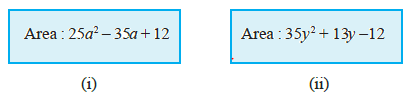Solution
(i) Area: $25a^2- 35a + 12$

Now $Area= Length \times Breadth$
So, factorizing Area
$25a^2- 35a + 12$
By Split middle method $=25a^2- 15a -20a + 12$
$= 5a (5a - 3) - 4(5a - 3)$
$=(5a - 4) (5a - 3)$
Possible expression for length= $5a - 4$
Possible expression for breadth=$5a - 3$

(ii)Area: $35y^2+ 13y- 12$
$35 y^2+ 13y - 12$
By Split middle method $=35y^2- 15y + 28y - 12$
$= 5y (7y - 3) + 4(7y - 3)$
$=(5y+ 4) (7y - 3)$
Possible expression for length=$(5y+ 4)$
Possible expression for breadth=$(7y - 3)$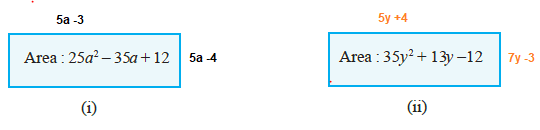Question 16
What are the possible expressions for the dimensions of the cuboids whose volumes are given below?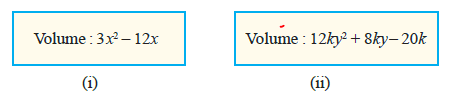(i) Volume: $3x^2- 12x$
$\text {Volume of Cuboids}= Length \times Breadth \times height$
Now, $3x^2- 12x$
$= 3x (x - 4)$
Possible expression for length=$3$
Possible expression for breadth=$x$
Possible expression for height=$(x - 4)$

(ii) Volume: $12ky^2+8ky -20k$
$\text {Volume of Cuboids}= Length \times Breadth \times height$
Now,
$12ky^2+ 8ky - 20k$
$= 4k (3y^2+ 2y - 5)$
$= 4k (3y^2+5y - 3y - 5)$
$=4k [y (3y +5) - 1(3y + 5)]$
$=4k (3y +5) (y - 1)$
Possible expression for length= $4k$
Possible expression for breadth=$(3y +5)$
Possible expression for height=$(y - 1)$

## Summary

1. NCERT Solutions for Class 9 Maths Chapter 2 Polynomial Exercise 2.4 and 2.5 has been prepared by Expert with utmost care. If you find any mistake.Please do provide feedback on mail. You can download the solutions as PDF in the below Link also
2. This chapter 2 has total 6 Exercise 2.1 ,2.2,2.3 ,2.4 and 2.5. This is the First exercise in the chapter.You can explore previous exercise of this chapter by clicking the link below